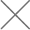mtbf计算方法计算实例

6000台产品,每天工作10小时,工作一个月后有6台失效,MTBF是多少？

1000台产品,每天工作24小时,工作一个月后有0.5台失效,MTBF是多少？

mtbf计算方法公式如下：

1Fit=1/10^9 小时 意为：10^9 小时坏了1台，失效率为1Fit

（1） 6000台10小时30天=1800000台时，一个月内坏了6台λ=6/180W

MTBF=180W/6=30W小时

（2） 1000台24小时30天=720000台时，一个月内坏了0.5台λ=0.5/72W

MTBF=72W/0.5=144W小时

（3） 6/180==2.4/72

n= 6000
t*= 300
r= 6

0.9990005 R(t)=e-λt
MTBF= 300000

n= 1000
t*= 720
r= 0.5
R（t)= 0.9995 =(1000-0.5)/1000
0.999500125 R(t)=e-λt
MTBF= 1440000

n= 1000
t*= 720
r= 2.4
R（t)= 0.9976
0.997602878
MTBF= 300000

NTEK北测检测，专业第三方可靠性检测机构，作为国家级高新技术企业、新型材料及电子产品综合公共测试平台，是中国第三方检测认证服务的开拓者，也是国内最早从事商品检验、鉴定、测试、认证等服务的专业机构之一，独立第三检测、测试、认证机构，多种检测设备，资深测试、认证工程师，为客户提供一站式检测、测试、认证服务。

•微信公众号

•微信咨询